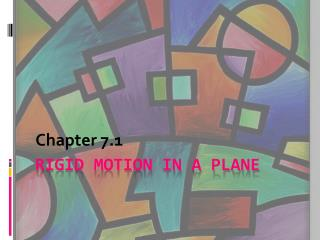DownloadDownload PresentationRigid Motion in a Plane

# Rigid Motion in a Plane

Download Presentation## Rigid Motion in a Plane

- - - - - - - - - - - - - - - - - - - - - - - - - - - E N D - - - - - - - - - - - - - - - - - - - - - - - - - - -
##### Presentation Transcript

1. Chapter 7.1 Rigid Motion in a Plane

2. New Vocabulary… • Pre Image – The original figure in the transformation of a figure in a plane. • Image – The new figure that results from the transformation of a figure in a plane.

3. New Vocabulary… • Transformation – The operation that maps, or moves, a pre image onto an image. • 3 basic transformations are reflections, rotations, and translations.

5. Example…

6. Mapping: A way to describe a transformation. • ABC is mapped on DEF • ABC DEF

7. Isometry: A transformation that preserves lengths. Also called a Rigid Transformation.

8. Chapter 7.2 Reflections

9. New Vocabulary… • Reflection – A type of transformation that uses a line that acts like a mirror, called a line of reflection, with an image reflected in the line. • Line m is the Line of Reflection. • Reflections are a type of Isometry. m

10. Reflections… Rules • If P is not on line m, then m is the perpendicular bisector of PP’. • If P is on m, then P = P’. m m P P P’ P’

11. Reflections… Rules Continued • If (x, y) is reflected in the x-axis, its image is the point (x, -y). • If (x, y) is reflected in the y-axis, its image is the point (-x, y). Reflect the GREEN dot over the y-axis. What are the coordinates of the pre image and image??? Reflect the PINK dot over the x-axis. What are the coordinates of the pre image and image???

12. Line of Symmetry…